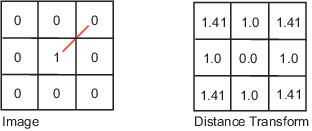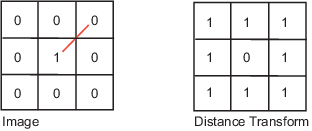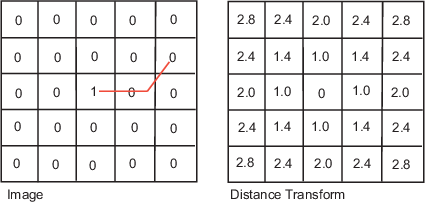Main Content

## Distance Transform of a Binary Image

The distance transform provides a metric or measure of the separation of points in the image. The `bwdist` function calculates the distance between each pixel that is set to `off` (`0`) and the nearest nonzero pixel for binary images.

The `bwdist` function supports several distance metrics.

Distance Metrics

Distance Metric

Description

Illustration

Euclidean

The Euclidean distance is the straight-line distance between two pixels.City Block

The city block distance metric measures the path between the pixels based on a 4-connected neighborhood. Pixels whose edges touch are 1 unit apart; pixels diagonally touching are 2 units apart.Chessboard

The chessboard distance metric measures the path between the pixels based on an 8-connected neighborhood. Pixels whose edges or corners touch are 1 unit apart.Quasi-Euclidean

The quasi-Euclidean metric measures the total Euclidean distance along a set of horizontal, vertical, and diagonal line segments.This example creates a binary image containing two intersecting circular objects.

```center1 = -10; center2 = -center1; dist = sqrt(2*(2*center1)^2); radius = dist/2 * 1.4; lims = [floor(center1-1.2*radius) ceil(center2+1.2*radius)]; [x,y] = meshgrid(lims(1):lims(2)); bw1 = sqrt((x-center1).^2 + (y-center1).^2) <= radius; bw2 = sqrt((x-center2).^2 + (y-center2).^2) <= radius; bw = bw1 | bw2; figure imshow(bw)```

To compute the distance transform of the complement of the binary image, use the `bwdist` function. In the image of the distance transform, note how the centers of the two circular areas are white.

```D = bwdist(~bw); figure imshow(D,[])```Download ebook